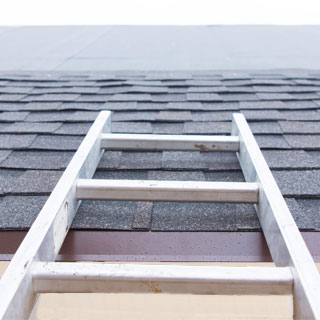The do it yourself resource for homeowners from home improvement professionals.

# How to Calculate Roof Area

If you’re in need of a new roof and want to find out how much it’ll cost, you can calculate the area of it, then use this number to estimate the cost of shingles per square foot. Here, our roofing installation experts share two ways for how to calculate roof area:

## What you’ll need to calculate roof area:• Large level
• Measuring tape
• Marker
• Pencil, paper

### Measuring the Pitch

To calculate the area of your roof, first you’ll need to calculate the pitch of it:

1. First, use your measuring tape to measure 12 inches on your large level and make a mark at the 12-inch line.
3. Climb to the top of your roof.
4. After that, place your level against the gable trim and flat against the side of the house.
5. Using your measuring tape, measure from the 12-inch mark on your level up to the bottom edge of your gable trim. This number is your rise.
6. Each rise measurement is written as “# in 12” and this is your roof’s pitch. (For example, if your rise is 4 inches, your roof’s pitch is 4 in 12.)

### Calculating the Area (Estimated)

If you don’t want to do a lot of measuring, you can use your pitch calculation to get an estimated square footage of your roof:

1. Once you have your roof’s pitch, divide the number by 12. (For example, if your roof’s pitch is 4 in 12, you would divide 4 by 12. This would yield 1/3.)
2. Next, square your result. (If your number is 1/3, squared would yield 1/9.)
3. After that, add 1 to your number. (1/9 + 1 = 10/9.)
4. Next, figure out the square root of your new number. (The square root of 10/9 is 1.05.)
5. Determine the square footage of one floor of your home. (For example, if you have a two-story home that’s 2,600 square feet, one floor would be 1,300 square feet.)
6. Multiply this number by your most recent result to get the estimated square footage of your roof. (1,300 x 1.05 = 1,365 square feet.)

### Calculating the Area (True)

If you want to get the exact area measurement for your roof, you can follow these steps:

1. Once you have your roof’s pitch, divide the number by 12. (For example, if your roof’s pitch is 4 in 12, you would divide 4 by 12. This would yield 1/3.)
2. Next, square your result. (If your number is 1/3, squared would yield 1/9.)
3. After that, add 1 to your number. (1/9 + 1 = 10/9.)
4. Next, figure out the square root of your new number. (The square root of 10/9 is 1.05.)
5. Next, use your measuring tape to measure the length of your house. (Be sure to include overhangs.)
6. After that, measure the width of your house. (Be sure to include overhangs.)
7. Multiply your house length by your house width to get the area. (For example, 40 feet x 30 feet = 1,200 square feet.)
8. Next, multiply the area by your roof’s pitch. (1,200 x 1.05 = 1,260 square feet.)
9. To allow for hips, ridges, and waste, add 10% of your final number for a gable roof and 17% of your final number for a cottage roof. (Your total number would be either 1,386 or 1,474 square feet.)

## Need help with this project?

### P.J. Fitzpatrick

21 Industrial Blvd
New Castle, DE 19720
1-877-246-4354

6380 Flank Dr #600
Harrisburg, PA 17112